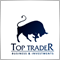291

i want add signal comment to chart in mql4  every 1 hr

```void OnTick()
{
//---
string symbols="";
***if(iVolume(NULL,timeframe,0)<=1){

for(int i=0; i<SymbolsTotal(true); i++)
{
int digits=MarketInfo(Symbol(),MODE_DIGITS);
double purplemagicline=iCustom(SymbolName(i,true),timeframe,"",2,1);
purplemagicline=NormalizeDouble(purplemagicline,digits);

double blueline=iCustom(SymbolName(i,true),timeframe,"",1,1);
blueline=NormalizeDouble(blueline,digits);

double redline=iCustom(SymbolName(i,true),timeframe,"",3,1);
redline=NormalizeDouble(redline,digits);

double greenline=iCustom(SymbolName(i,true),timeframe,"",4,1);
greenline=NormalizeDouble(greenline,digits);

if((----))
if((--))
symbols+=SymbolName(i,true)+"\n";
}

}
Comment("\n",symbols);

}```

this code

but it comment A moment and Fade.

I want my code to be reviewed once a hour and not commented on20808

1. ```         if((----))
if((--))
```
Don't post code that won't even compile.

2. `    ***if(iVolume(NULL,timeframe,0)<=1){`

For a new bar test, Bars is unreliable (a refresh/reconnect can change number of bars on chart,) volume is unreliable (miss ticks,) Price is unreliable (duplicate prices and The == operand. - MQL4 programming forum.) Always use time.

I disagree with making a new bar function, because it can only be called once per tick. A variable can be tested multiple times.
New candle - MQL4 programming forum

3. Saeid Reza Bahman Abadi: but it comment A moment and Fade.
Of course, it does. What does your code do when it is not the start of a new bar?
```void OnTick(){
string symbols="";
Comment("\n",symbols);
}```